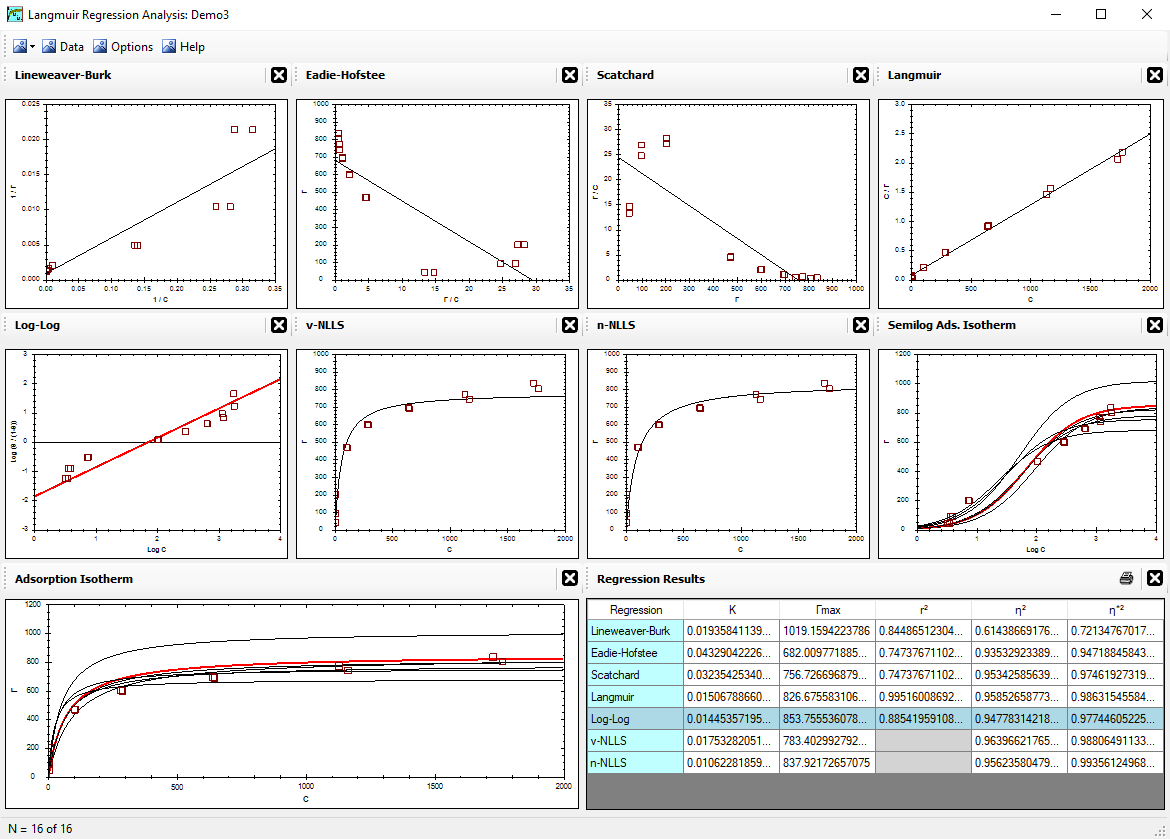Alfisol, Item 003: LMMpro, version 2.0 The Langmuir Optimization Program plus The Michaelis-Menten Optimization Program## NLLS Regressions

With computers, it is rather easy to optimize a parabolic equation using nonlinear methods. The optimization minimizes the squares of the error terms, and hence these methods are known as Nonlinear Least Squares (NLLS) methods. There are two NLLS methods used by LMMpro: one that minimizes the vertical error (v-NLLS), the other minimizes the normal error (n-NLLS). The equation parameters are incrementally changed until a minimum in the sum-of-the-squares-of-the-errors is attained.

Using v-NLLS, the error is defined as the square of the vertical distance between each datum point and the predicted curve.

Using n-NLLS, the error is defined as the square of the normal distance between each datum point and the predicted curve. The normal distance is defined as the perpendicular distance from the nearest place on the curve.When using n-NLLS, the slope of the error line between the datum point and the predicted curve will change depending on the location of the datum point. It will be nearly horizontal in the lower left corner of the graph. It will be nearly vertical in the upper right corner of the graph. Accordingly, with n-NLLS you must decide upon the relative importance of a horizontal error versus a vertical error. A diagonal error line will have both vertical error and horizontal error components. The ACF value is used to determine the relative impact of these types of error.

The Axes Conversion Factor (ACF) describes the relative importance (weight) of an error in x versus an error in y. IF ACF = 1, then x-axis and y-axis values have the same weight. A large ACF value (>1) means that the y-axis values are weighed more strongly. A small ACF value (<1) means that the x-axis values are weighed more strongly.

NLLS Regression Bias

The v-NLLS method is biased toward optimizing the fit with the data in the lower left side of the graph. This is because this area of the graph will generate large errors if the predicted curve is not properly tracking these data. Notice that even a small change in the predicted curve will slightly shift the location of the sharp rise in the parabolic equation, which, in turn, results in a large vertical error with each datum point in that area.

Using the n-NLLS method, a small change in the predicted curve will not result in any significant increase in its distance to any of the data points. Accordingly, the n-NLLS method is not biased toward optimizing the fit with the data in any particular area of the graph.

The n-NLLS regression method used by LMMpro was presented by Schulthess & Dey in 1996 (Soil Sci. Soc. Am. J. 60:433-442).